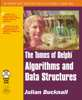www.boyet.com
 HomeBook's main page

Tomes of Delphi:
Algorithms and Data Structures

This page describes the errata for Chapter 4 of Tomes of Delphi: Algorithms and Data Structures (DADS).

Errata for Chapter 4: Searching

1. Page 130. Despite proofreading this several times, the fact that I'm assuming throughout that L can be greater than R (it can't) completely eluded me. I state that L could have been equal to R+1 in the final loop. No, this is false; it could have been equal to R-1: I made the subtraction an addition. So, here's how the start of this argument should read:

As it happens, it can. Look back at the implementation of binary search for the array in Listing 4.9. When we drop out of the bottom of the loop, having determined that the item could not be found, what can we deduce about the values of L, R, and M? Firstly, and most obviously, L > R. Consider now the final cycle through the loop. At the start of this cycle, we must have had L = R, or L = R-1, and M would have been calculated as equal to L. If there were any greater gap between L and R, say L = R-2, then M would have fallen in between them and we would have been able to go round the loop at least once more.

Thanks to Nico Schoemaker.

Copyright (c) Julian M Bucknall, 2001 Last modified: 02-May-2002. email: Webmaster